# Bipolar Junction Transistors in Active Mode

## Discrete Semiconductor Devices and Circuits

• #### Question 1

 Don’t just sit there! Build something!!

Learning to mathematically analyze circuits requires much study and practice. Typically, students practice by working through lots of sample problems and checking their answers against those provided by the textbook or the instructor. While this is good, there is a much better way.

You will learn much more by actually building and analyzing real circuits, letting your test equipment provide the “answers” instead of a book or another person. For successful circuit-building exercises, follow these steps:

1. Carefully measure and record all component values prior to circuit construction, choosing resistor values high enough to make damage to any active components unlikely.
2. Draw the schematic diagram for the circuit to be analyzed.
3. Carefully build this circuit on a breadboard or other convenient medium.
4. Check the accuracy of the circuit’s construction, following each wire to each connection point, and verifying these elements one-by-one on the diagram.
5. Mathematically analyze the circuit, solving for all voltage and current values.
6. Carefully measure all voltages and currents, to verify the accuracy of your analysis.
7. If there are any substantial errors (greater than a few percent), carefully check your circuit’s construction against the diagram, then carefully re-calculate the values and re-measure.

When students are first learning about semiconductor devices, and are most likely to damage them by making improper connections in their circuits, I recommend they experiment with large, high-wattage components (1N4001 rectifying diodes, TO-220 or TO-3 case power transistors, etc.), and using dry-cell battery power sources rather than a benchtop power supply. This decreases the likelihood of component damage.

As usual, avoid very high and very low resistor values, to avoid measurement errors caused by meter “loading” (on the high end) and to avoid transistor burnout (on the low end). I recommend resistors between 1 kΩ and 100 kΩ.

One way you can save time and reduce the possibility of error is to begin with a very simple circuit and incrementally add components to increase its complexity after each analysis, rather than building a whole new circuit for each practice problem. Another time-saving technique is to re-use the same components in a variety of different circuit configurations. This way, you won’t have to measure any component’s value more than once.

• #### Question 2

Models of complex electronic components are useful for circuit analysis, because they allow us to express the approximate behavior of the device in terms of ideal components with relatively simple mathematical behaviors. Transistors are a good example of components frequently modeled for the sake of amplifier circuit analysis: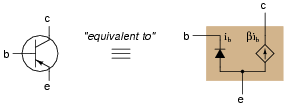It must be understood that models are never perfect replicas of the real thing. At some point, all models fail to precisely emulate the thing being modeled. The only real concern is how accurate we want our approximation to be: which characteristics of the component most concern us, and which do not.

For example, when analyzing the response of transistor amplifier circuits to small AC signals, it is often assumed that the transistor will be “biased” by a DC signal such that the base-emitter diode is always conducting. If this is the case, and all we are concerned with is how the transistor responds to AC signals, we may safely eliminate the diode junction from our transistor model: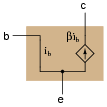However, even with the 0.7 volt (nominal) DC voltage drop absent from the model, there is still some impedance that an AC signal will encounter as it flows through the transistor. In fact, several distinct impedances exist within the transistor itself, customarily symbolized by resistors and lower-case r′ designators: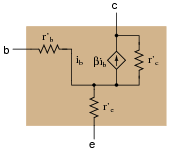From the perspective of an AC current passing through the base-emitter junction of the transistor, explain why the following transistor models are equivalent: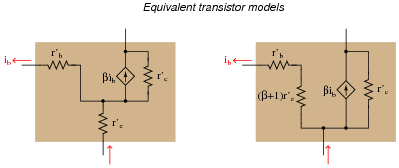• #### Question 3

Load lines are useful tools for analyzing transistor amplifier circuits, but they may be hard to understand at first. To help you understand what “load lines” are useful for and how they are determined, I will apply one to this simple two-resistor circuit: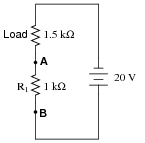We will have to plot a load line for this simple two-resistor circuit along with the “characteristic curve” for resistor R1 in order to see the benefit of a load line. Load lines really only have meaning when superimposed with other plots. First, the characteristic curve for R1, defined as the voltage/current relationship between terminals A and B: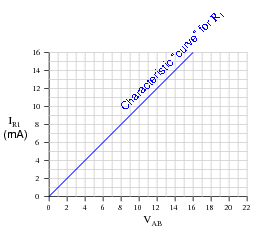Next, I will plot the load line as defined by the 1.5 kΩ load resistor. This “load line” expresses the voltage available between the same two terminals (VAB) as a function of the load current, to account for voltage dropped across the load: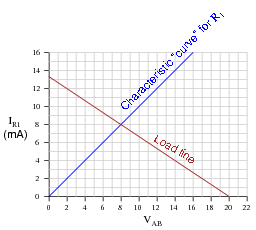At what value of current (IR1) do the two lines intersect? Explain what is significant about this value of current.

• #### Question 4

Load lines are useful tools for analyzing transistor amplifier circuits, but they may be applied to other types of circuits as well. Take for instance this diode-resistor circuit:The diode’s characteristic curve is already plotted on the following graph. Your task is to plot the load line for the circuit on the same graph, and note where the two lines intersect: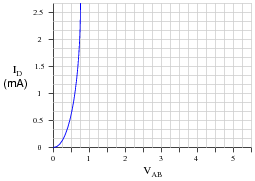What is the practical significance of these two plots’ intersection?

• #### Question 5

A very important measure of a transistor’s behavior is its characteristic curves, a set of graphs showing collector current over a wide range of collector-emitter voltage drops, for a given amount of base current. The following plot is a typical curve for a bipolar transistor with a fixed value of base current: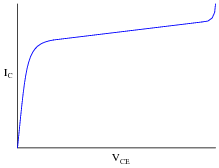A “test circuit” for collecting data to make this graph looks like this:Identify three different regions on this graph: saturation, active, and breakdown, and explain what each of these terms mean. Also, identify which part of this curve the transistor acts most like a current-regulating device.

• #### Question 6

If a transistor is subjected to several different base currents, and the collector-emitter voltage (VCE) ßwept” through the full range for each of these base current values, data for an entire “family” of characteristic curves may be obtained and graphed: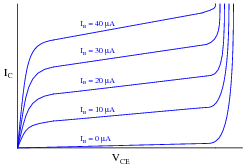What do these characteristic curves indicate about the base current’s control over collector current? How are the two currents related?

• #### Question 7

Conduction of an electric current through the collector terminal of a bipolar junction transistor requires that minority carriers be “injected” into the base region by a base-emitter current. Only after being injected into the base region may these charge carriers be swept toward the collector by the applied voltage between emitter and collector to constitute a collector current: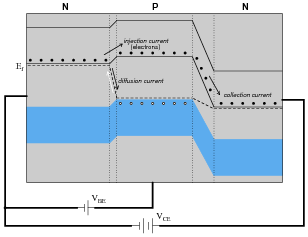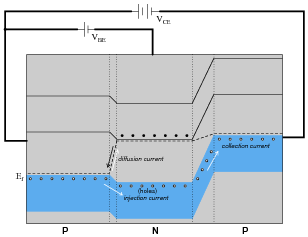An analogy to help illustrate this is a person tossing flower petals into the air above their head, while a breeze carries the petals horizontally away from them. None of the flower petals may be “swept” away by the breeze until the person releases them into the air, and the velocity of the breeze has no bearing on how many flower petals are swept away from the person, since they must be released from the person’s grip before they can go anywhere.

By referencing either the energy diagram or the flower petal analogy, explain why the collector current for a BJT is strongly influenced by the base current and only weakly influenced by the collector-to-emitter voltage.

• #### Question 8

Determine the approximate amount of collector current for this transistor circuit, given the following characteristic curve set for the transistor: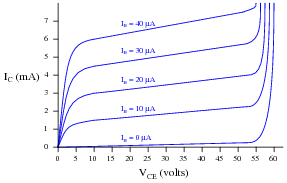• #### Question 9

The following schematic diagram is of a simple curve tracer circuit, used to plot the current/voltage characteristics of different electronic components on an oscilloscope screen:The way it works is by applying an AC voltage across the terminals of the device under test, outputting two different voltage signals to the oscilloscope. One signal, driving the horizontal axis of the oscilloscope, represents the voltage across the two terminals of the device. The other signal, driving the vertical axis of the oscilloscope, is the voltage dropped across the shunt resistor, representing current through the device. With the oscilloscope set for “X-Y” mode, the electron beam traces the device’s characteristic curve.

For example, a simple resistor would generate this oscilloscope display: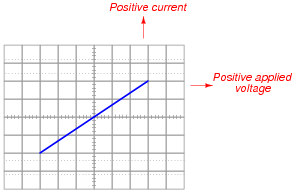A resistor of greater value (more ohms of resistance) would generate a characteristic plot with a shallower slope, representing less current for the same amount of applied voltage: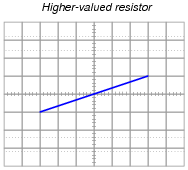Curve tracer circuits find their real value in testing semiconductor components, whose voltage/current behaviors are nonlinear. Take for instance this characteristic curve for an ordinary rectifying diode: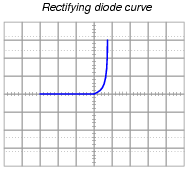The trace is flat everywhere left of center where the applied voltage is negative, indicating no diode current when it is reverse-biased. To the right of center, though, the trace bends sharply upward, indicating exponential diode current with increasing applied voltage (forward-biased) just as the “diode equation” predicts.

On the following grids, plot the characteristic curve for a diode that is failed shorted, and also for one that is failed open: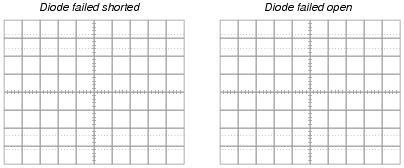• #### Question 10

Explain why a bipolar junction transistor tends to regulate collector current over a wide range of collector-to-emitter voltage drops when its base current is constant. What happens internally that makes the BJT’s collector current relatively independent of collector-to-emitter voltage and strongly dependent on base current?

• #### Question 11

Many technical references will tell you that bipolar junction transistors (BJTs) are current-controlled devices: collector current is controlled by base current. This concept is reinforced by the notion of “beta” (β), the ratio between collector current and base current:

$$\beta = \frac{I_C}{I_B}$$

Students learning about bipolar transistors are often confused when they encounter datasheet specifications for transistor β ratios. Far from being a constant parameter, the “beta” ratio of a transistor may vary significantly over its operating range, in some cases exceeding an order of magnitude (ten times)!

Explain how this fact agrees or disagrees with the notion of BJTs being “current-controlled” devices. If collector current really is a direct function of base current, then why would the constant of proportionality between the two (β) change so much?

• #### Question 12

A common term used in semiconductor circuit engineering is small signal analysis. What, exactly, is ßmall signal” analysis, and how does it contrast with large signal analysis?

• #### Question 13

Explain what it means for a transistor to operate in its “active” mode (as opposed to cutoff, saturation, or breakdown).

• #### Question 14

We know that graphs are nothing more than collections of individual points representing correlated data in a system. Here is a plot of a transistor’s characteristic curve (for a single value of base current):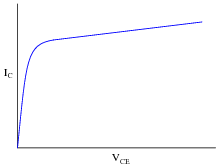And here is a plot of the “load line” for a transistor amplifier circuit: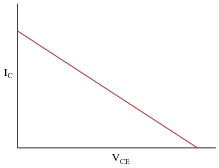For each of these graphs, pick a single point along the curve (or line) and describe what that single point represents, in real-life terms. What does any single point of data along either of these graphs mean in a transistor circuit?

If a transistor’s characteristic curve is superimposed with a load line on the same graph, what is the significance of those two plots’ intersection?

• #### Question 15

Describe what a load line is, at it appears superimposed on this graph of characteristic transistor curves: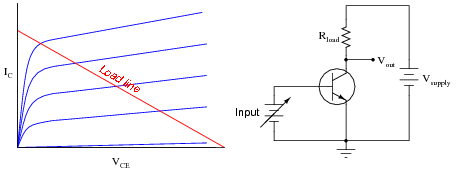What exactly does the load line represent in the circuit?

• #### Question 16

Though the characteristic curves for a transistor are usually generated in a circuit where base current is constant and the collector-emitter voltage (VCE) is varied, this is usually not how transistor amplifier circuits are constructed. Typically, the base current varies with the input signal, and the collector power supply is a fixed-voltage source:The presence of a load resistor in the circuit adds another dynamic to the circuit’s behavior. Explain what happens to the transistor’s collector-emitter voltage (VCE) as the collector current increases (dropping more battery voltage across the load resistor), and qualitatively plot this load line on the same type of graph used for plotting transistor curves:• #### Question 17

Calculate and superimpose the load line for this circuit on top of the transistor’s characteristic curves: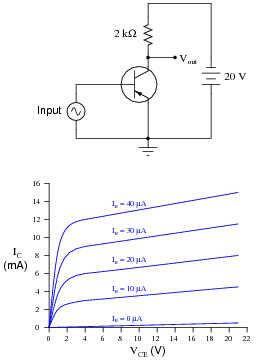Then, determine the amount of collector current in the circuit at the following base current values:

IB = 10 μA
IB = 20 μA
IB = 30 μA
IB = 40 μA
• #### Question 18

In this graph you will see three different load lines plotted, representing three different values of load resistance in the amplifier circuit: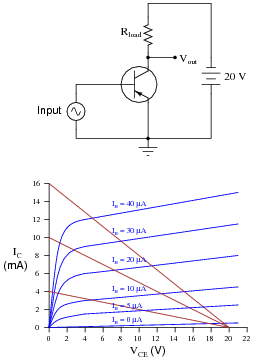Which one of the three load lines represents the largest value of load resistance (Rload)? Which of the three load lines will result in the greatest amount of change in voltage drop across the transistor (∆VCE) for any given amount of base current change (∆IB)? What do these relationships indicate about the load resistor’s effect on the amplifier circuit’s voltage gain?

• #### Question 19

An important parameter of transistor amplifier circuits is the Q point, or quiescent operating point. The “Q point” of a transistor amplifier circuit will be a single point somewhere along its load line.

Describe what the “Q point” actually means for a transistor amplifier circuit, and how its value may be altered.

• #### Question 20

The following graph is a family of characteristic curves for a particular transistor: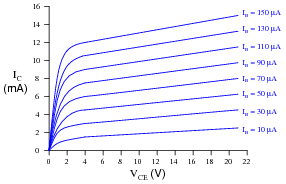Draw the load line and identify the Q-point on that load line for a common-collector amplifier circuit using this transistor:• #### Question 21

The following graph is a family of characteristic curves for a particular transistor:Draw the load line and identify the Q-point on that load line for a common-emitter amplifier circuit using this transistor:• #### Question 22

The following graph is a family of characteristic curves for a particular transistor:Superimpose on that graph a load line for the following common-emitter amplifier circuit using the same transistor:Also determine some bias resistor values (R1 and R2) that will cause the Q-point to rest approximately mid-way on the load line.

R1 = R2 =

• #### Question 23

Find one or two real bipolar junction transistors and bring them with you to class for discussion. Identify as much information as you can about your transistors prior to discussion:

Terminal identification (which terminal is base, emitter, collector)
Continuous power rating
Typical β